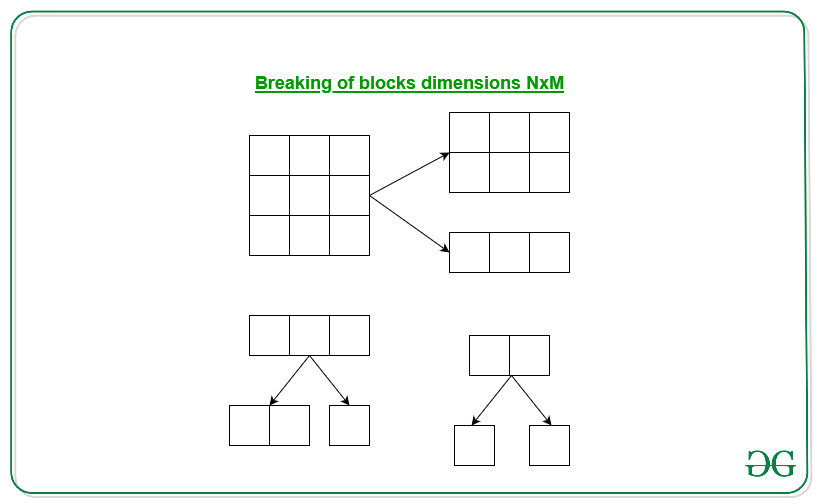# Identical Splitting in a rectangular grid

• Difficulty Level : Hard
• Last Updated : 30 Sep, 2022

Given a rectangular grid NxM dimensions, the task is to find the minimum number of cuts required to break the given rectangular grid into a square of size 1×1.

Examples:

Input: N = 4, M = 4
Output: 15

Input: N = 2, M = 1
Output:

Approach:The above images shows the splitting of the rectangular grid. We can observe that every single cut increases the number of rectangles of different dimensions by 1. We will do the splitting until we reach the square of dimension 1×1.
So for the given rectangular dimensions of NxM, the total number of squares of dimensions 1×1 is N*M. Therefore we required N*M – 1 cuts to break the given rectangular dimensions of NxM into squares of dimension 1×1.

Below is the implementation of the above approach:

## C++

 `// C++ program of the above approach``#include ``using` `namespace` `std;`` ` `// Function to find the minimum cuts``void` `minimumCuts(``int` `N, ``int` `M)``{`` ` `    ``// Print the minimum cuts using``    ``// the formula``    ``cout << (N * M - 1);``}`` ` `// Driver Code``int` `main()``{`` ` `    ``// Given dimensions``    ``int` `N = 4, M = 4;`` ` `    ``// Function call``    ``minimumCuts(N, M);`` ` `    ``return` `0;``}`

## Java

 `// Java program of the above approach``import` `java.util.*;`` ` `class` `GFG{`` ` `// Function to find the minimum cuts``static` `void` `minimumCuts(``int` `N, ``int` `M)``{``     ` `    ``// Print the minimum cuts using``    ``// the formula``    ``System.out.print(N * M - ``1``);``}`` ` `// Driver Code``public` `static` `void` `main(String[] args)``{``     ` `    ``// Given dimensions``    ``int` `N = ``4``, M = ``4``;`` ` `    ``// Function call``    ``minimumCuts(N, M);``}``}`` ` `// This code is contributed by Rohit_ranjan`

## Python3

 `# Python3 program of the above approach`` ` `# Function to find the minimum cuts``def` `minimumCuts(N, M):``     ` `    ``# Print the minimum cuts using``    ``# the formula``    ``print``(N ``*` `M ``-` `1``)`` ` `# Driver Code ``if` `__name__ ``=``=` `"__main__"``:``     ` `    ``# Given dimensions``    ``N ``=` `4``    ``M ``=` `4``     ` `    ``# Function call``    ``minimumCuts(N, M)``     ` `# This code is contributed by coder001`

## C#

 `// C# program of the above approach``using` `System;`` ` `class` `GFG{`` ` `// Function to find the minimum cuts``static` `void` `minimumCuts(``int` `N, ``int` `M)``{``     ` `    ``// Print the minimum cuts using``    ``// the formula``    ``Console.Write(N * M - 1);``}`` ` `// Driver Code``public` `static` `void` `Main(String[] args)``{``     ` `    ``// Given dimensions``    ``int` `N = 4, M = 4;`` ` `    ``// Function call``    ``minimumCuts(N, M);``}``}`` ` `// This code is contributed by Princi Singh`

## Javascript

 ``

Output:

`15`

Time Complexity: O(1)

My Personal Notes arrow_drop_up